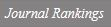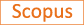Existence and multiplicity of solutions for equations of p(x)-laplace type in rN without AR-condition

Title
Existence and multiplicity of solutions for equations of p(x)-laplace type in rN without AR-condition
Authors
Kim J.-M.Kim Y.-H.Lee J.
Ewha Authors
Issue Date
2018
Journal Title
Differential and Integral Equations
ISSN
0893-4983Citation
Differential and Integral Equations vol. 31, pp. 435 - 464
Publisher
Khayyam Publishing
Indexed
SCIE; SCOPUSDocument Type
Article
Abstract
We are concerned with the following elliptic equations with variable exponents −div(ϕ(x,∇u)) + V(x)

u

p(x)−2u = λf(x,u) in RN, where the function ϕ(x,v) is of type

v

p(x)−2v with continuous function p : RN → (1,∞), V : RN → (0,∞) is a continuous potential function, and f : RN×R → R satisfies a Carathéodory condition. The aims of this paper are stated as follows. First, under suitable assumptions, we show the existence of at least one nontrivial weak solution and infinitely many weak solutions for the problem without the Ambrosetti and Rabinowitz condition, by applying mountain pass theorem and fountain theorem. Second, we determine the precise positive interval of λ’s for which our problem admits a nontrivial solution with simple assumptions in some sense. © 2018 Khayyam Publishing. All Rights Reserved.
Appears in Collections:
연구기관 > 수리과학연구소 > Journal papers
Files in This Item:
There are no files associated with this item.
Export
RIS (EndNote)
XLS (Excel)
XML